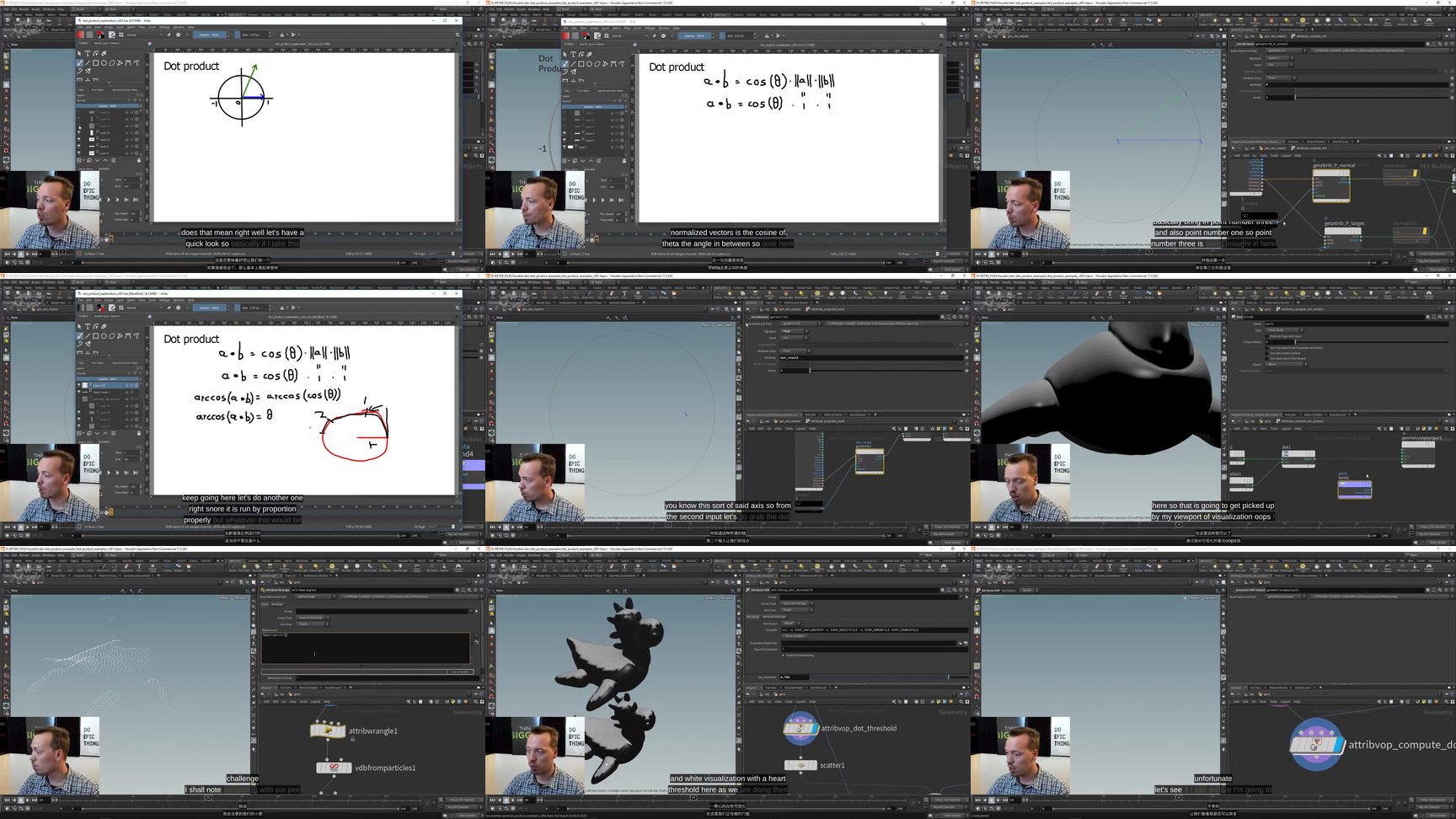# 理解什么是点乘Understanding the DOT product!

Dot product wikipedia: http://bit.ly/WikiDot
Dot product on Khan Academy: http://bit.ly/KhanDot

FIRST: Geometric definition of the dot product.
Finding information on wikipedia and Khan Academy.
Geometric definition of the dot product:
a · b = |a| × |b| × cos(theta)

SECOND: Range of the dot product.
Range of the dot product is -1 to 1. The dot project is the result of one vector projected onto another vector. Why do you need the dot product? There are some really useful applications for the dot product: Find out if two vectors align, are perpendicular to each other, are opposite to each other. We can cover objects with snow or find out which way is facing the sun. We can also use it for Field of View as part of crowds or a flocking system.

THIRD: Example in Houdini.
Working with normalized vectors simplifies the mathematics.
When the angle between the vectors is 0, then the dot product will be a value of 1. When the angle is 90, the dot product will be 0. When the angle is 180 degrees, then the dot product will be a value of -1.

FOURTH: Computing the angle in between – theory.
The dot product is represented with a big dot.
General equation: a · b = |a| × |b| × cos(theta)
After normalizing a and b: a · b = 1 × 1 × cos(theta)
Simplifies to: a · b = cos(theta)
To get the angle theta we can apply the arccos: arccos(a · b) = arccos(cos(theta))
This simplifies to: arccos(a · b) = theta

FIFTH: Creating the demo example.
Creating various line segments to represent the two vectors. The target vector traces a circle defined by the sin(\$FF*5) in the y-axis and cos(\$FF*5) in the z-axis. Showing how to normalize the line segment that represents the ‘target’ vector.
Next we build the ‘normal’ segment that lies on the z-axis.

SIXTH: computing the dot product.
Next we compute the dot product inside an attribute vop. We can use a ‘get attribute’ to grab the positions of the end points (that represent the normals) of both the ‘target’ segment and the ‘normal’ segment. We compute the dot product with a ‘dot product’ vop and output it into a ‘dot_result’.

SEVENTH: Computing the angle in between – implementation.
Divide the dot product by the multiplication of the length of the vectors will correctly scale the dot product before computing the angle. If one or both vectors have a value of zero, than the dot product will not be calculated correctly. The result of the division feeds into the arccos calculation which results in the angle in radians.

Mapping one ‘radius length’ along the circumference of the circle represents 1 radian. 180 degrees represents pie radians. 360 degrees represents 2*pie*r radians, which is also the definition of the circumference of the circle. Convert the angle theta from radians to degrees.

NINTH: Rounding the dot and angle values.
Round the dot and angle to 3 values behind the period. Reference the dot_result_rounded_string in the Font sop so we can see it in the viewport. Remaining edge segments are polywired and merged with the font sops. We also compute the projected point onto the z-axis, resample the projected line segment, delete every other line segment with a modulo operation.

TENTH: Cover rubber toy with snow.
We want to identify the surface normals that are a certain amount in alignment with the y-axis. Inside of an attribute vop we compute the dot product between the normalized surface normal N and the normalized user specified direction vector. We normalize the resulting dot product by fitting(x,-1,1,0,1). This gives us a useful gradient.

ELEVENTH: Isolate the top area of the mesh.
Inside of an attribute vop we can do a comparison between a hard threshold specified by the user and the result of the dot product calculation. This will allow us to isolate the top polygons on the mesh. Next we scatter some points on the white polygons.

TWELFTH: Converting scattered points to snow volume.
Using vdb from particles we turn the points into a sdf volume. If the pscale is too small we need to lower the voxel size to capture the small scale details. To smooth the sdf we use a ‘vdb smooth sdf’ sop. We use an attribute wrangle to specify the pscale for all points.

THIRTEENTH: Dot product restrictions and workarounds.
The dot product only does normal projection, it can not take (self-)occlusion into account. Instead we can use the ray-sop to project points on the mesh.

FOURTEENTH: Group by normal & dot against sun.
The group by normal makes use of the dot product algorithm underneath. A primitive sphere represents the position of the sun. Compute the difference vector between the surface point and the sun target point. Next compute the dot product between the surface normal and the difference vector.

For business or one-on-one visual effects consulting inquiries, you can reach me at peterclaesbusiness@gmail.com

Dot product wikipedia: http://bit.ly/WikiDot
Dot product on Khan Academy: http://bit.ly/KhanDot

a·b = | a | ×| b | ×cos（theta）

SEVENTH：计算两者之间的角度 – 实施。

NINTH：舍入点和角度值。

TENTH：用雪覆盖橡胶玩具。

ELEVENTH：隔离网格的顶部区域。

TWELFTH：将散乱点转换为雪量。

For business or one-on-one visual effects consulting inquiries, you can reach me at peterclaesbusiness@gmail.com### 2 说点什么1 评论条数
1 评论回复
0 订阅者

2 评论者最近评论者
想得到该评论有新回复的通知？